Digital Logic

For two binary variables (taking values 0 and 1) there are 16 possible functions. The functions involve only three operations which make up Boolean algebra: AND, OR, and COMPLEMENT. They are symbolically represented as follows: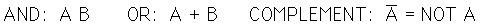These operations are like ordinary algebraic operations in that they are commutative, associative, and distributive. There is a group of useful theorems of Boolean algebra which help in developing the logic for a given operation.

 Digital Logic Theorems Digital Logic Functions
Index

Electronics concepts

Digital Circuits

 HyperPhysics*****Electricity and magnetism R Nave
Go Back

Boolean Algebra Theorems

The applications of digital logic involve functions of the AND, OR, and NOT operations. These operations are subject to the following identities: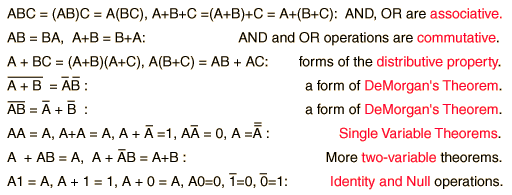These theorems can be used in the algebraic simplification of logic circuits which come from a straightforward application of a truth table.

 DeMorgan's Theorem
 Basic Gates
Index

Electronics concepts

Digital Circuits

 HyperPhysics*****Electricity and magnetism R Nave
Go Back

Binary Functions of Two Variables

Digital logic involves combinations of the three types of operations for two variables: AND, OR, and NOT. There are sixteen possible functions:

This is an active graphic. Click on any of the functions for further details.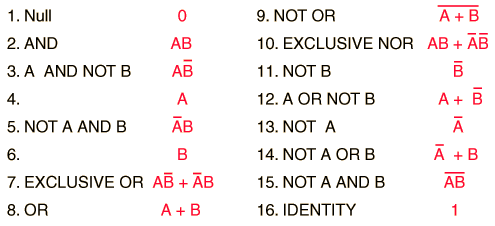Index

Electronics concepts

Digital Circuits

 HyperPhysics*****Electricity and magnetism R Nave
Go Back

Single Variable Theorems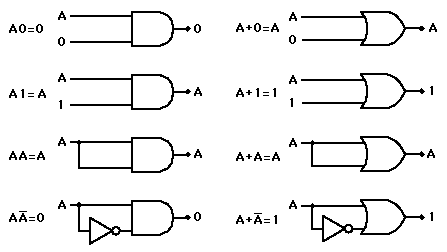Digital Logic Theorems Digital Logic Functions
Index

Electronics concepts

Digital Circuits

 HyperPhysics*****Electricity and magnetism R Nave
Go Back

Two-Variable Theorems

Besides the important DeMorgan's Theorem, the theorems below have utility in digital circuits. They have no direct counterparts in ordinary algebra.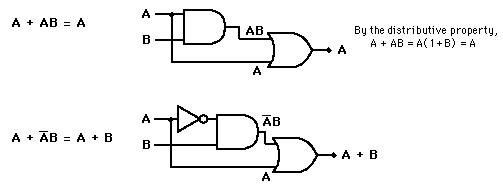Digital Logic Theorems Digital Logic Functions
Index

Electronics concepts

Digital Circuits

 HyperPhysics*****Electricity and magnetism R Nave
Go Back